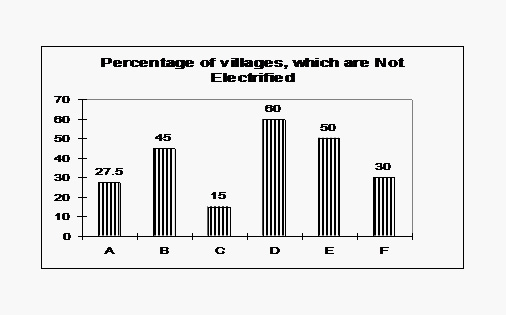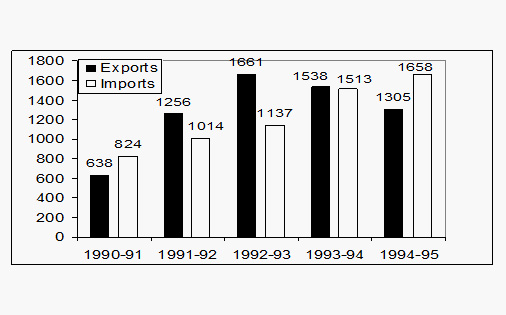# Bar Graph Questions: Level 01

DIRECTIONS for questions 1 - 5: Study the following graph carefully and answer the questions given below:1. If the Central Govt. desires to give funds for speedy electrification, which state will get 3rd rank in the order of priority?
A. C
B. B
C. A
D. None of these
2. Which state has three times the percentage of non- electrified villages in comparison to 'C' state?
A. C
B. F
C. B
D. None of these
3. In case of State B, what percent of villages are electrified?
A. 75%
B. 15%
C. 45%
D. None of these
4. How many states have atmost 40% or less non electrified villages?
A. 5
B. 3
C. 4
D. 6
5. Which state has the minimum % of Non electrified villages?
A. A
B. B
C. C
D. D
DIRECTIONS for questions 6 to 10: Study the following graph carefully and answer the questions given below:1. What is the ratio of exports in years 1990-91 & 1992-93 together to the imports in the same year?
A. 1.41
B. 1.17
C. 1.55
D. 1.71
2. In which of the following years was the gap in the exports and imports the maximum?
A. 1994-95
B. 1992-93
C. 1993-94
D. 1990-91
3. What is the ratio of years having above average imports to those having below average imports in the given period?
A. 3:2
B. 4:1
C. 2:3
D. 1:4
4. Which of the following statements is true about the graph?
A. There is a continuous rise in exports.
B. From 1992-93, exports are below average.
C. Till 1992-93, imports are below average.
D. Total imports in the given period are more than the total exports.
5. The exports in 1993-94 are approximately how many percent more than the imports of 1991-92?
A. 49%
B. 51.6%
C. 201%
D. None of these